# Olympic Games Costs

Costs of the Olympic Games held from 1960 to 2014

## Examples

### Basic Examples

Retrieve the resource:

 In:=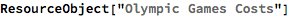Out=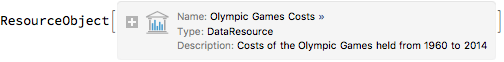Retrieve the default content:

 In:=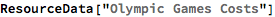Out=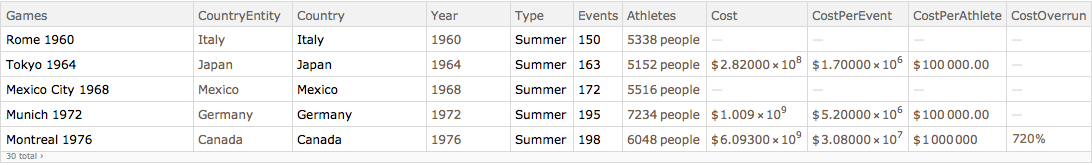### Visualization

See which countries have spent the most to host the Olympic Games:

 In:=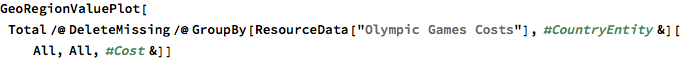Out=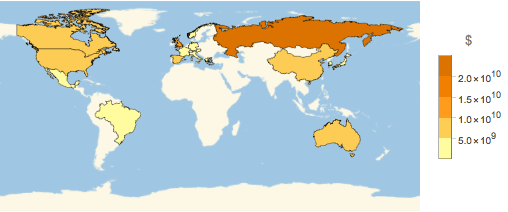Plot costs per event over time:

 In:=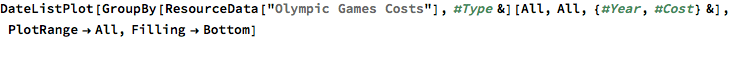Out=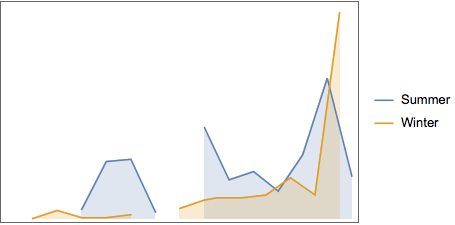Plot the countries that have hosted both Winter and Summer Olympics:

 In:=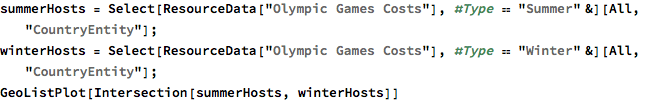Out=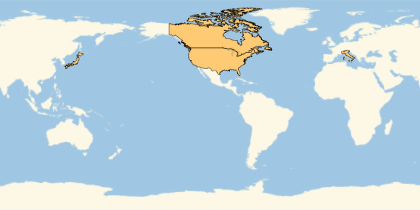See which countries have hosted the most Olympic Games:

 In:=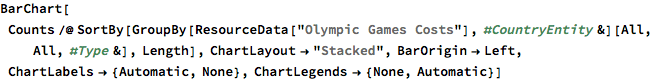Out=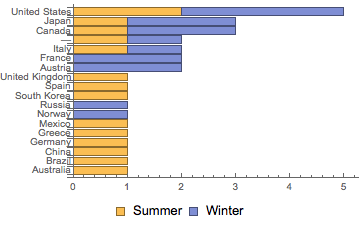Wolfram Research, "Olympic Games Costs" from the Wolfram Data Repository (2017) https://doi.org/10.24097/wolfram.79874.data